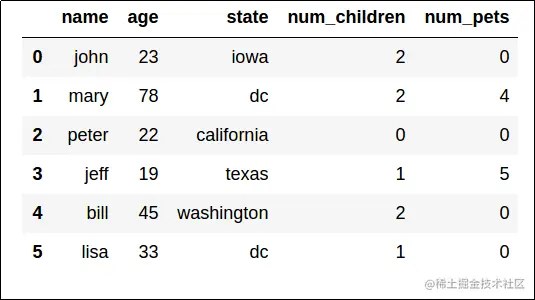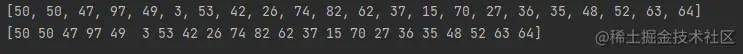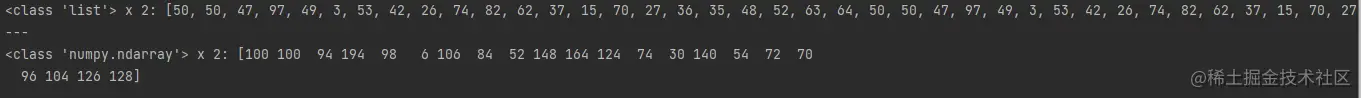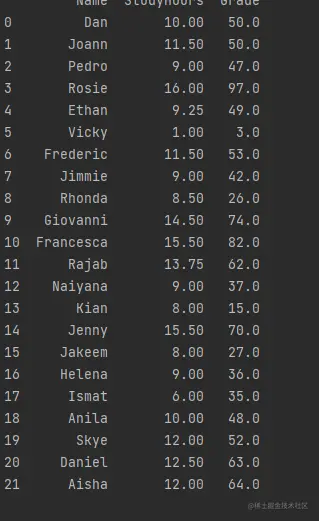# Getting started exploring and analyzing data using Python

2022-02-02 03:57:20

Tutorial from docs.microsoft.com/ Learn as much as you can

• # Use Python Explore and analyze data

After decades of open source development ,Python Provide rich functions through a powerful statistical and numerical library ：

• NumPy and Pandas Simplify data analysis and operation
• Matplotlib Provide compelling data visualization
• Scikit-learn Provide simple and effective predictive data analysis
• TensorFlow and PyTorch Provide machine learning and deep learning functions

# utilize NumPy and Pandas Browsing data

Data scientists can use a variety of tools and techniques to browse 、 Visualize and manipulate data . One of the most common ways for data scientists to process data is to use Python Language and some specific data processing packages .

## What is? NumPy

NumPy It's a Python library , Provide with MATLAB and R And other mathematical tools . Even though NumPy Greatly simplifies the user experience , But it also provides a comprehensive mathematical function .

## What is? Pandas

Pandas Is an extremely popular Python library , For data analysis and operation . Pandas about Python It's like excel, Provide easy-to-use features for data tables .## Explore Jupyter The data in the notebook

Jupyter Notebook It's using Web A common way for browsers to run basic scripts . Usually , These notebooks are single web pages , Decompose into on the server （ Not the local computer ） The text part and code part executed on . This means you can start quickly , Without installation Python Or other tools .

## Test hypothesis

Data exploration and analysis is usually an iterative process , In which data scientists sample data , And perform the following tasks to analyze the data and test the hypothesis ：

• Clean up data to handle errors 、 Missing values and other problems .
• Apply statistical techniques to better understand data , Better understand how the sample is expected to represent the overall data of the real world （ Allow random variation ）.
• Visually present the data to determine the relationship between variables , In machine learning projects , Identify features that may predict the label .
• Revise the assumptions and repeat the process .

Use NumPy Explore data arrays

Let's look at some simple data first .

Suppose a University collects a sample of student scores for a data science course .

``````data = [50,50,47,97,49,3,53,42,26,74,82,62,37,15,70,27,36,35,48,52,63,64]
print(data)
Copy code ``````

Data loaded into Python In the list structure , This is a good data type for general data operations , But there is no optimization for numerical analysis . So , We will use NumPy package , It includes Python Use in Numbers Specific data types and functions .

``````import numpy as np
Copy code ``````If you want to know the list and NumPy The difference between arrays , Let's compare these data types by multiplying... In the expression 2 The performance of time .Please note that , Multiply the list by 2 A new list will be created that is twice the length of the original list element sequence . On the other hand , take NumPy Array multiplication performs calculation by element , The behavior of the array is similar to vector, So you end up with an array of the same size , Each of these elements is multiplied by 2.
The key lies in NumPy Arrays are specially designed to support mathematical operations of numerical data —— This makes them more useful in data analysis than generic lists .

``````grades.shape
Copy code ``````

Confirm that the array has only one dimension , It includes 22 Elements ( There are... In the original list 22 A score ). You can access each element in the array by its zero - based ordinal position . Let's get the first element ( Location 0 the ). Okay , Now you are familiar with NumPy Array , It's time to do some analysis of the performance data . You can apply aggregation across elements in an array , So let's find a simple average score `grades.mean()` 49.18181818181818 So his average score is 49 branch Let me add another set of data Let's add a second set of data for the same students , This time, record the typical hours they spend studying every week

``````study_hours = [10.0,11.5,9.0,16.0,9.25,1.0,11.5,9.0,8.5,14.5,15.5,
13.75,9.0,8.0,15.5,8.0,9.0,6.0,10.0,12.0,12.5,12.0]

# Create a 2D array (an array of arrays)
student_data = np.array([study_hours, grades])

# display the array
print(student_data)
Copy code ``````

# Show a two-dimensional array

print(student_data.shape) Now it becomes a two-dimensional array Ahead is the study time Then there are the results Used to analyze relevant information

# Get the average value of each sub column

``````avg_study = student_data.mean()

print('Average study hours: {:.2f}\nAverage grade: {:.2f}'.format(avg_study, avg_grade))
Copy code ``````

## Use Pandas Explore tabular data

although NumPy Provides many functions required to process numbers , Especially numeric arrays ; When you start working with a two-dimensional data table ,Pandas The package provides a more convenient structure - DataFrame.

Run the following cells to import Pandas Library and create a three column DataFrame. The first column is a list of student names , The second and third columns contain data on study time and achievement NumPy Array .

``````import pandas as pd

df_students = pd.DataFrame({'Name': ['Dan', 'Joann', 'Pedro', 'Rosie', 'Ethan', 'Vicky', 'Frederic', 'Jimmie',
'Rhonda', 'Giovanni', 'Francesca', 'Rajab', 'Naiyana', 'Kian', 'Jenny',
'Jakeem','Helena','Ismat','Anila','Skye','Daniel','Aisha'],
'StudyHours':student_data,
Copy code ``````### stay DataFrame Find and filter data in

You can use DataFrame Of loc Method to retrieve data for a specific index value , As shown below .

### The index is 5 The data of

`df_students.loc`

### There are also slicing operations

`df_students.loc[0:5]`

Except that it can be used loc Method to find out from the row according to the index , You can also use it iloc The method is based on the line DataFrame in Find the row at the ordinal position of （ Index independent ）：
go through `iloc[0:5]` result , And connect them with `loc[0:5]` Compare your previous results . Can you see the difference ？

Described LOC Method returns rows and indexes label The values in the list 0 to 5, It includes - 0,1,2,3,4, and 5（6 That's ok ）. however ,iloc Method returns the range 0 To 5 It contains In position The line of , And because the integer range does not include the upper limit value , This includes location 0123 and 4（ Five elements ） .

iloc adopt Location identification DataFrame Medium Data values , Location Expand from row to column . therefore , for example , You can use it to find the number 0 Position in row 1 and 2 The value of the column in , As shown below ： `df_students.iloc[0,[1,2]]`

Let's go back to loc Method , See how it handles Columns . please remember ,loc Used to locate data items based on index values rather than locations . Without explicitly indexed columns , The rows in our data frame are indexed to integer values , But the column is identified by name ： `df_students.loc[0,'Grade']`

This is another useful technique . You can use loc Method finds the index row based on the filter expression , The expression refers to a named column other than the index , As shown below ：`df_students.loc[df_students['Name']=='Aisha']`

In order to better measure , You can use DataFrame Of Inquire about Method to get the same result , As shown below ：`df_students[df_students['Name']=='Aisha']`

The previous three examples emphasize the use of Pandas Sometimes confusing facts . Usually , There are several ways to achieve the same result . Another example is when you quote DataFrame How column names are . You can specify column names as named index values （ Such as `df_students['Name']` The example we've seen so far ）, Or you can use this column as DataFrame Properties of , As shown below ：`df_students[df_students.Name == 'Aisha']`

### Load from file DataFrame

We built... From some existing arrays DataFrame. however , In many actual scenarios , Data is loaded from sources such as files . Let's replace the student grade with the content of the text file DataFrame.

``````df_students = pd.read_csv('grades.csv',delimiter=',',header='infer')
Copy code ``````

DataFrame Of read_csv Method to load data from a text file . As you can see in the sample code , You can specify such as column delimiters and which row （ If there is ） Contains column headings （ under these circumstances , The separator is a comma , The first row contains column names —— These are the default settings , So you can omit the parameter ）.

### Handling missing values

One of the most common problems that data scientists need to deal with is incomplete or missing data . So how do we know DataFrame Including missing values ？ You can use isnull Method to identify which individual values are null, As shown below ： `df_students.isnull()` Of course , For the larger DataFrame, It would be inefficient to view all rows and columns individually ; So we can get the sum of the missing values in each column , As shown below ：`df_students.isnull().sum()` So now we know that there is a lack of StudyHours Values and two are missing Grade value .

To view them in context , We can filter the data frame to contain only any columns （ The axis of the data frame 1） Empty lines . `df_students[df_students.isnull().any(axis=1)]` retrieval DataFrame when , Missing values are displayed as NaN Not numbers ）.

So now that we have found the null value , What can we do with them ？

A common method is Estimate Replacement value . for example , If you lack study hours , We can assume that students learn the average time and replace the missing value with the average learning hours . So , We can use fillna Method , As shown below ： `df_students.StudyHours = df_students.StudyHours.fillna(df_students.StudyHours.mean())` perhaps , It may be important to ensure that you only use data that you know is absolutely correct ; So you can use dropna Method to delete a row or column containing a null value . under these circumstances , We will delete any row whose column contains null values （DataFrame The shaft 0）`df_students = df_students.dropna(axis=0, how='any')`

### Explore DataFrame Data in

Now we have cleaned up the missing values , We are ready to explore DataFrame Data in . Let's start by comparing average study time and grades .

``````mean_study = df_students['StudyHours'].mean()

# Get the mean grade using the column name as a property (just to make the point!)

# Print the mean study hours and mean grade
print('Average weekly study hours: {:.2f}\nAverage grade: {:.2f}'.format(mean_study, mean_grade))
Copy code ``````

well , Let's filter DataFrame To find only students who spend more time than average .

`df_students[df_students.StudyHours > mean_study]` Please note that , The filtered result itself is a DataFrame, So you can handle it like any other DataFrame Treat its columns the same way .

for example , Let's find out the average score of students who study more than the average study time . `df_students[df_students.StudyHours > mean_study].Grade.mean()`

Let's assume that the passing grade for the course is 60.

We can use this information to DataFrame Add a new column , Indicate whether each student has passed .

First , We'll create a file that contains / Failure indicators （True or False） Of Pandas series , Then we connect the series as DataFrame A new column in （ Axis 1）. `passes = pd.Series(df_students['Grade'] >= 60) df_students = pd.concat([df_students, passes.rename("Pass")], axis=1)` DataFrames Designed for tabular data , You can use them to perform a variety of data analysis operations that you can perform in a relational database ; For example, grouping and aggregating data tables .

for example , You can use groupby Method is based on the... You added earlier Pass Columns group student data , Count the number of names in each group - let me put it another way , You can determine how many students pass and fail . `print(df_students.groupby(df_students.Pass).Name.count())`

You can aggregate multiple fields in a group using any available aggregation function . for example , You can find the average learning time and grades of the student groups who passed and failed the course `print(df_students.groupby(df_students.Pass)['StudyHours', 'Grade'].mean())`

DataFrame It's very versatile , You can easily manipulate data . many DataFrame Operation return DataFrame New copy of ; So if you want to modify one DataFrame But keep the existing variables , You need to assign the result of the operation to an existing variable . for example , The following code divides the student data into Grade null , And the sorted DataFrame Assigned to the original df_students Variable . `df_students = df_students.sort_values('Grade', ascending=False)`

Numpy and DataFrames yes Python The main force of Data Science . They provide us with loading 、 Methods of exploring and analyzing tabular data . As we will see in the following modules , Even advanced analysis methods often rely on Numpy and Pandas To play these important roles .